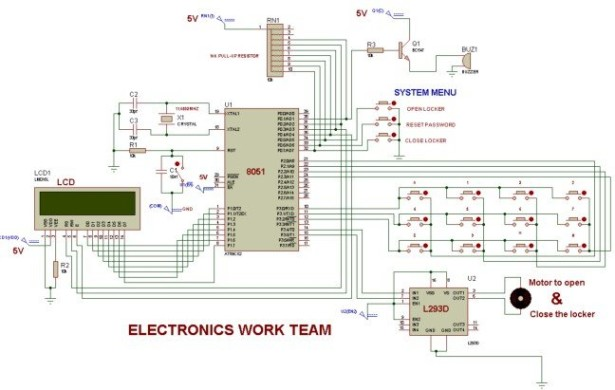# 8051 MICROCONTROLLER

## 8051 Microcontroller based electronic locker system

CIRCUIT DESCRIPTION

Security is a prime concern in our day-today life. Everyone wants to be as much secure as possible.The issue of security is very paramount at home doors and safe. An access control for doors formsvital.link
in a security chain. Therefore we intend to aid in security at home by bringing in an electronic code lock system that involves an individual to enter the password before getting an access to some items, a particular room or building.This code lock system is not just the normal single user code lock system that required a user to insert an already programmed code to gain access to a room or safe; it is a code lock system that has password and enable multiple user access.

The microcontroller based Door locker is an access control system that allows only authorized persons to access a restricted area. The system is fully controlled by the 8 bit microcontroller AT89C2051 which has a 2Kbytes of ROM for the program memory. The system has a Keypad by which the password can be entered through it. When the entered password equals with the password stored in the memory then the door is opened. If we entered a wrong password for more than three times then the Alarm is switched on.

The data pins of LCD are connected to P1 port & RS,R/W,E pins are connected to P0.2,P0.3,P0.4. L293D is used to rotate motor bidirectionaly to open & close the locker. A 4*3 keypad is used to enter the numbers 0 to 9 & ‘*’, ‘#’. There is a system menu, contains three buttons for open locker, reset password & close the locker.`PROJECT CODE`

#include< reg51.h >
#define col P2
#define row P3

sbit m1=P3^4;
sbit m2=P3^5;
sbit bu=P0^1;
sbit rs=P0^2;
sbit rw=P0^3;
sbit en=P0^4;
sbit start=P0^5;
sbit rst=P0^6;
sbit mp=P0^7;
sfr lcddata=0x90;

void delay(int a)
{
int i,j;
for(i=0;i<a;i++) for(j=”0;j<1100;j++);” }=”” void=”” command(unsigned=”” char=”” s)=”” initialise=”” of=”” the=”” lcd=”” {=”” lcddata=”s;” rs=”0;” rw=”0;” en=”1;” delay(5);=”” lcddisplaydata(unsigned=”” data=”” writing=”” delay(8);=”” displaydata(unsigned=”” *word)=”” int=”” x;=”” for(x=”0;word[x]!=0;x++)” lcddisplaydata(word[x]);=”” unsigned=”” array=”{‘0′,’1′,’2′,’3’,” ‘4’,’5′,’6′,’7′,=”” ‘8’,’9′,’*’,’#’};=”” n=”0;” m=”0;” i,p,q,r;=”” main()=”” colloc,=”” rowloc;=”” array1;=”” array2=”{‘3′,’1′,’1′,’2’};” array3;=”” locker1:=”” col=”0xFF;” row=”0x00;” m1=”0;” m2=”0;” bu=”0;” mp=”1;” p=”0;” q=”0;” r=”0;” start=”1;” rst=”1;” command(0x01);=”” command(0x38);=”” command(0x0c);=”” command(0x84);=”” displaydata(“press=”” key”);=”” command(0xc0);=”” displaydata(“from=”” system=”” menu”);=”” while(1)=”” if(start=”=0)” goto=”” locker2;=”” else=”” if(rst=”=0)” locker3;=”” locker2:=”” command(0x82);=”” delay(10);=”” displaydata(“enter=”” your”);=”” command(0xc3);=”” displaydata(“password”);=”” delay(80);=”” command(0x80);=”” locker5;=”” locker3:=”” current”);=”” command(0xc4);=”” locker4:=”” command(0x83);=”” new”);=”” displaydata(”=”” password”);=”” locker5:=”” do=”” colloc=”col;” &=”0x0f;” }while(colloc!=”0x0f);” }while(colloc=”=0x0f);” if(colloc!=”0x0f)” rowloc=”0;” break;=”” if(p=”=1)” if(colloc=”=0x0E)” array1[n]=”array[rowloc];” n++;=”” locker6;=”” if(q=”=1)” locker7;=”” if(r=”=2)” locker11;=”” array2[n]=”array[rowloc];” locker9;=”” array3[n]=”array[rowloc];” locker8;=”” locker6:=”” if(n<4)=”” lcddisplaydata(‘*’);=”” if(n=”=4)” delay(50);=”” if(array1=”=array2&&array1==array2&&array1==array2&&array1==array2)” displaydata(“right=”” locker14;=”” displaydata(“wrong=”” locker15;=”” locker7:=”” locker4;=”” locker12;=”” locker8:=”” if(array3=”=array2&&array3==array2&&array3==array2&&array3==array2)” displaydata(“new=”” password=”” has”);=”” displaydata(“been=”” set”);=”” delay(100);=”” locker1;=”” locker13;=”” locker9:=”” locker10;=”” locker10:=”” displaydata(“conform=”” locker11:=”” locker16;=”” locker12:=”” m++;=”” if(m<3)=”” delay(500);=”” locker13:=”” locker14:=”” delay(125);=”” while(mp!=”0);” locker15:=”” locker16:=”” <=””>

## How to interface LEDs with 8051 Microcontroller

#### Current Project / Post can also be found using:

• 8051 microcontroller
• anything
• microcontroller 8051
• microcontroller 8051 modulation